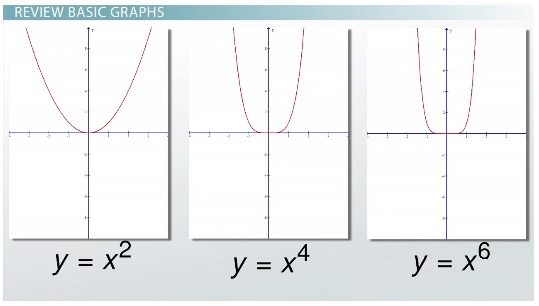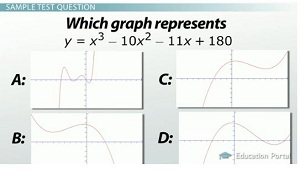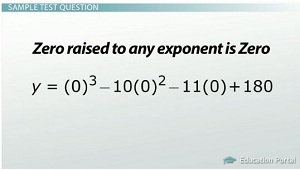# How to Graph Cubics, Quartics, Quintics and Beyond

An error occurred trying to load this video.

Try refreshing the page, or contact customer support.

Coming up next: How to Add, Subtract and Multiply Polynomials

### You're on a roll. Keep up the good work!

Replay
Your next lesson will play in 10 seconds
• 0:06 Review Basic Graphs
• 1:26 Local Maximum and Minimums
• 3:43 Sample Test Question
• 9:50 Sketching For Class
• 10:14 Lesson Summary

Want to watch this again later?

Timeline
Autoplay
Autoplay
Speed

#### Recommended Lessons and Courses for You

Lesson Transcript

Chad has taught Math for the last 9 years in Middle School. He has a M.S. in Instructional Technology and Elementary Education.

Finding the graph of a polynomial isn't too hard. If you can graph, you already know the major steps! With a couple rules and helpful memorizing tools, you will be graphing polynomials in no time!

## Review Basic Graphs

In this lesson, we're looking at how to graph several equations that contain different exponential values. Let's start by reviewing the graphs of y = x^2, y = x^4 and y = x^6. We notice that the graphs with positive leading coefficients and even exponents have an end behavior - that is, the ends of their graphs go up to the left and up to the right.Next, let's look at what happens when our graphs have negative coefficients in front. In this screen, we see the graphs of y = -x^2, y = -x^4 and y = -x^6. If the leading coefficient is negative, their end behavior is opposite, so it will go down to the left and down to the right.

Let's continue our review with odd exponents. Here you see the graphs of y = x^3, y = x^5 and y = x^7. We notice that the graphs with positive leading coefficients and odd exponents have an end behavior going down to the left and up to the right. As we add a negative sign in front of our leading coefficient, we see that our graphs change. If the leading coefficient is negative, their end behavior goes up to the left and down to the right.

## Local Maximum and Minimums

The previous graphs were all simple forms of a more complex graphing process. Most of the graphs we will look at have a whole bunch of hills and valleys called local maximums and local minimums. To graph an equation that is more complex, we will need to know where the hills and valleys occur. A relative local maximum is the highest bump in a graph; we can think of this as a hill. A relative local minimum is the lowest bump on the graph; we can think of this as a valley.

Let's see what these type of graphs look like. Let's look at the graph of y = x^3 + 3x^2 - 16x - 48. As you can see, the highest bump in the graph is our local max, or hill. It isn't our maximum point because the graph goes up to infinity on the end of our graph here. So it is our local max. We can also notice local minimum, or the valley. The minimum is not an absolute minimum because the graph goes down to negative infinity here.

This equation can also give us tips about how many bumps we could find in the graph. To calculate how many bumps any equation will have, we take the degree of the equation and subtract one. The degree of the equation is the largest exponential value found in the equation.Let's look at the equation y = x^3 + 3x^2 - 16x - 48. The degree of this equation is 3. To find out how many bumps we can find, we take the degree of the equation and subtract one: 3 - 1 = 2. So at most we would find two bumps in our graph. Now we're ready to start the fun part - graphing without a calculator using what we know about graphing polynomials. On a multiple-choice test, we aren't going to literally graph the equation; we are going to pick the answer that shows the graph. With what we have learned, this should be fairly easy.

## Sample Test Question

Let's look at a sample test question. Which graph represents the equation y = x^3 - 10x^2 - 11x + 180? Looking at these four multiple choice options, let's use what we know about graphing polynomials to select the correct answer. To begin, let's graph what we know about this equation on a blank Cartesian plane.

We know that our graph will go down to the left and up to the right because the leading coefficient, 1, is positive and its exponential power of 3 is odd. Answer choice B can be eliminated because it goes up to the left and down to the right.We can also determine that there will be two bumps on the graph because the degree power of the equation is 3. To calculate the number of bumps, remember we subtract the degree power of the equation minus 1. So, 3 - 1 = 2 bumps. So the maximum number of bumps we will find in this equation will be two. So right away we can cancel out any graph that has more than two bumps. We can cancel out graph A because it has more than two bumps.

We are now left with two answer choices: C and D. The next way that we can determine which graph matches the equation is to find the y-intercept. To find the y-intercept, we will need to evaluate the equation for x = 0.

Starting with our equation, y = x^3 - 10x^2 - 11x + 180, we will plug in a zero for every x value we see. Now our equation looks like y = (0)^3 - 10(0)^2 - 11(0) + 180. To solve this equation, we will first do our exponents. Zero raised to any exponent is zero. Our equation now looks like y = 0 - 10(0) - 11(0) + 180. Next, we need to do all of the multiplication. Again, any number times zero equals zero. Our equation simplifies to y = 180. So our y-intercept for this equation is (0, 180).

It looks like the graphs of answer choices C and D both intersect the y-axis at (0, 180). The y-intercept does not help us in this case pick our correct answer. Let's graph some other points that we can find on a blank Cartesian plane to see which graph best matches the correct answer.

To unlock this lesson you must be a Study.com Member.

### Register to view this lesson

Are you a student or a teacher?

### Unlock Your Education

#### See for yourself why 30 million people use Study.com

##### Become a Study.com member and start learning now.
Back
What teachers are saying about Study.com

### Earning College Credit

Did you know… We have over 160 college courses that prepare you to earn credit by exam that is accepted by over 1,500 colleges and universities. You can test out of the first two years of college and save thousands off your degree. Anyone can earn credit-by-exam regardless of age or education level.